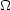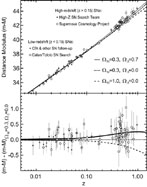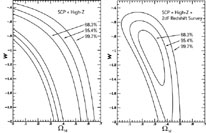5.3. High Redshift SNIa Observations

The SCP  in 1997 presented their first results with 7 objects at a redshift around z = 0.4. These objects hinted at a decelerating Universe with a measurement ofM = 0.88+0.69-0.60, but were not definitive. Soon after, the SCP published a further result, with a z ~ 0.84 SNIa observed with the KECK I and HST added to the sample , and the HZSNS presented the results from their first four objects [22, 87]. The results from both teams now ruled out aM = 1 Universe with greater than 95% significance. These findings were again superceded dramatically when both teams announced results including more SNe (10 more HZSNS SNe, and 34 more SCP SNe) that showed not only were the SN observations incompatible with aM = 1 Universe, they were also incompatible with a Universe containing only normal matter [77, 83]. Fig. 4 shows the Hubble diagram for both teams. Both samples show that SNe are, on average, fainter than would be expected, even for an empty Universe, indicating that the Universe is accelerating. The agreement between the experimental results of the two teams is spectacular, especially considering the two programs have worked in almost complete isolation from each other.Figure 4. Upper panel: The Hubble diagram for high redshift SNIa from both the HZSNS  and the SCP . Lower panel: The residual of the distances relative to aM = 0.3,= 0.7 Universe. The z < 0.15 objects for both teams are drawn from CTSS sample , so many of these objects are in common between the analyses of the two teams.

The easiest solution to explain the observed acceleration is to include an additional component of matter with an equation of state parameter more negative than w < - 1/3; the most familiar being the cosmological constant (w = - 1). Fig. 5 shows the joint confidence contours for values ofM andfrom both experiments. If we assume the Universe is composed only of normal matter and a cosmological constant, then with greater than 99.9% confidence the Universe has a non-zero cosmological constant or some other form of dark energy.Figure 5. The confidence regions for both HZSNS  and SCP  forM,. The two experiments show, with remarkable consistency, that> 0 is required to reconcile observations and theory. The SCP result is based on measurements of 42 distant SNIa. (The analysis shown here is uncorrected for host galaxy extinction;see  for the alternative analyses with host extinction correction, which is shown to make little difference in this data set.) The HZSNS result is based on measurements of 16 SNIa, including 6 snapshot distances , of which two are SCP SNe from the 42 SN sample. The z < 0.15 objects used to constrain the fit for both teams are drawn from the CTSS sample , so many of these objects are common between the analyses by the two teams.

Of course, we do not know the form of dark energy which is leading to the acceleration, and it is worthwhile investigating what other forms of energy are possible additional components. Fig. 6 shows the joint confidence contours for the HZSNS+SCP observations forM and wx (the equation of state of the unknown component causing the acceleration). Because this introduces an extra parameter, we apply the additional constraint thatM +x = 1, as indicated by the CMB experiments . The cosmological constant is preferred, but anything with a w < - 0.5 is acceptable [23, 77]. Additionally, we can add information about the value ofM, as supplied by recent 2dF redshift survey results , as shown in the 2nd panel, where the constraint strengthens to w < - 0.6 at 95% confidence .Figure 6. Left panel: Contours ofM versus wx from current observational data. Right Panel: Contours ofM versus wx from current observational data, where the current value ofM is obtained from the 2dF redshift survey. For both panelsM +x = 1 is taken as a prior.